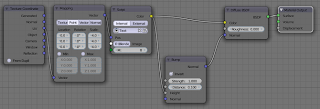### Chaos mosaic with OSL

Chaos mosaic is a technique tht can be used to reduce the repetative appearance of tilebale images. An example is shown below. The upper right plane shows visible repetition artifacts that are not apparent in the lower left plane.

(I used a 700x700 tileable texture from CGtextures.com (Gravel0170_3_S.jpg) repeated four times in each direction on the upper right plane, and the same image with the chaosmosaic shader on the lower left plane)

The shader code to implement this is very short:
```shader chaosmosaic(
point Pos=P,
string Image="",
int M=10,

output color Color=0
){
float x,y;

x=mod(Pos,1);
y=mod(Pos,1);

float xi=floor(x*M);
float yi=floor(y*M);

// mapping here
float xp=noise("cell",Pos,xi*M+yi);
float yp=noise("cell",Pos,xi*M+yi+M*M);

float xoffset = mod(mod(x,1.0/M)+xp,1);
float yoffset = mod(mod(y,1.0/M)+yp,1);
Color = texture(Image,xoffset,yoffset);
}```
In the code above M is the number of squares in each direction in our uv map. We use it to calculate two indices (xi, and yi) that are subsequently used pick a random position in the texture (xp,yp). The pixel from the texture is then selected according to the relative offset in the small square inside the uv map (xoffset, yoffset). The original algorithm also uses a blending function between adjacent tiles form the textures but that will blur the image. In this case we didn't implement that because from far enough away the small tile edges aren't noticable but the large scale repetion has gone away so with very little code we already have a better result.

## Example node setup

The node setup used for the example image (the plane on the lower left) looks like this:## reference

If you would like to know more about programming OSL you might be interested in my book "Open Shading Language for Blender". More on the availability of this book and a sample can be found on this page.

#### 1 comment:

1.Thanks a lot Michel! very useful your script!
I wish you a happy holiday season!
Bye bye.
Spirou4D